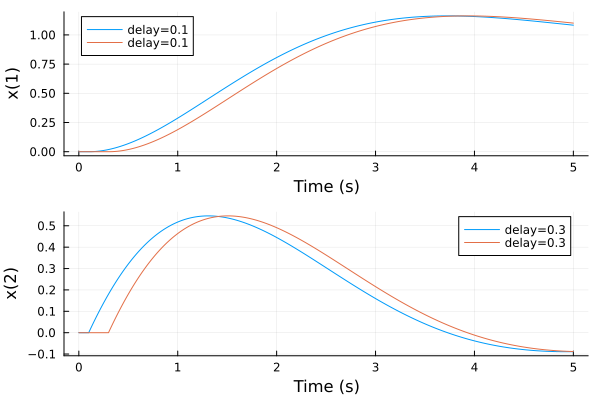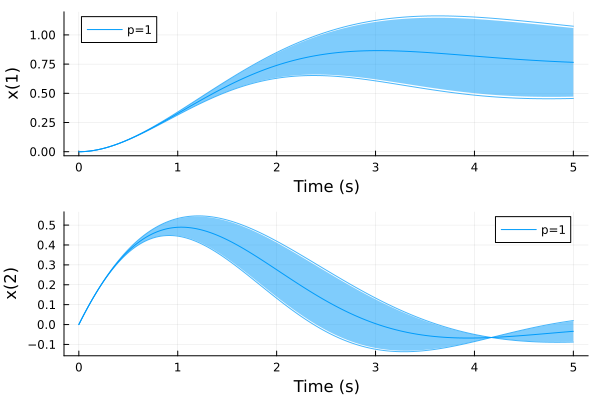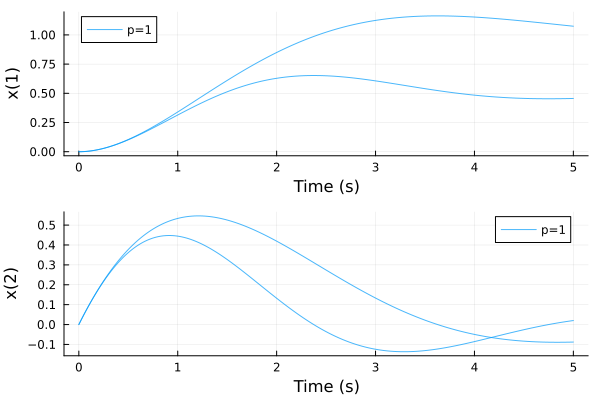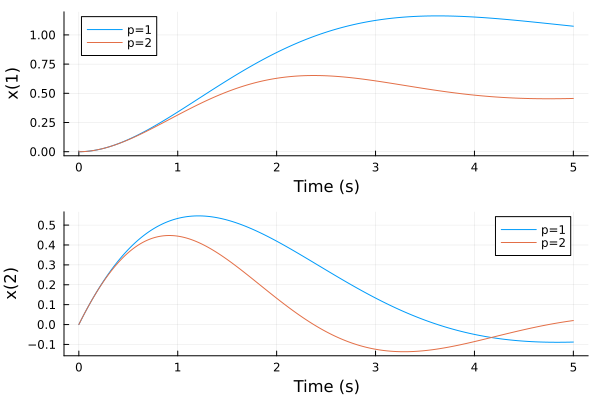# ControlSystems.jl Uncertain Model Parameters and Time Delays

I am using ControlSystems.jl with MonteCarloMeasurements.jl to analyse the step response of models with uncertain parameters and time delays. However, plotting the step response of a model with uncertain parameters vs. a model with uncertain time delays gives two different illustrations.

For example, running the following code,

``````sys = tf(1, [1, 1, 1]) * delay(Particles([0.1, 0.3]))
plot(step(sys, 0:0.01:5.0), lab=["delay=0.1" "delay=0.3"], ploty=false, plotx=true)
``````

displays the below figure:The above figure compares the step responses of the simple systems with time delays of 0.1s and 0.3s. I would like to produce the same plot but with two different model parameters (instead of time delays). However, the following code,

``````sys = tf(1, [1, 1, Particles([1, 2])])
plot(step(sys, 0:0.01:5.0), ploty=false, plotx=true)
``````

displays the below figure:Here the result looks like a ribbonplot from MonteCarloMeasurements.jl, where for the time delay uncertainty the figure looks like a mcplot.

My question is why are these two results different? Is there a way to change how each one is displayed? For my use case, I prefer the first plot (where each parameter is plotted as a separate line). Any insight anyone has is greatly appreciated!

I’m surprised the first one worked the way it did. It “should” look like the second one. Also, I can’t seem to reproduce the first due to an error I’m hitting in `lsim` internally, but that’s a separate issue.

One solution here, though, is to use the `array_of_structs` function from MonteCarloMeasurements.

``````using ControlSystems, MonteCarloMeasurements, Plots
const MCM = MonteCarloMeasurements

sys = tf(1, [1, 1, 1]) * delay(Particles([0.1, 0.3]))
sols = MCM.array_of_structs(x -> step(x, 0:0.01:5.0), sys)
plot(sols, lab=["delay=0.1" "delay=0.3"], ploty=false, plotx=true)
``````

``````sys = tf(1, [1, 1, Particles([1, 2])])
sols = MCM.array_of_structs(x -> step(x, 0:0.01:5.0), sys)
plot(sols, ploty=false, plotx=true)
``````

Not sure why the labels are on the wrong things, though.

1 Like

Thank you. That array of structs function is useful!

And yes, it would of made more sense to me if both my plots looked like the second example. I was only confused because they plotted so differently.

Cheers for the help.

1 Like

Since there are two series produced by each system, the label has to be repeated twice `lab=["0.1" "0.1" "0.3" "0.3"]`. I wish I had a better solution to thisGood question, I had thought both of them should look like the second one. I’ll have a look. Like Jonnie, I did get an error when using the first code you posted… once. After that it appears to workI’ll look into that as well.

Yes, there is a keywords to turn ribbon off: `ri = false`

2 Likes

The answer to why they are different, and why @jonniedie didn’t get the first one to work, is that it requires loading RobustAndOptimalControl, which has most of the functionality required to tie MCM and CS together (docs on uncertainty modeling). There is a special method to handle `lsim` with uncertain time delays which produces a vector of `SimResult` rather than a `SimResult` with particles. To be consistent, this method could potentially fold all results into a single results with `Particles`.

2 Likes

Ah thank you for that - I was unaware of this keyword. It doesn’t plot exactly how I would expect though:

``````using Plots, ControlSystems, MonteCarloMeasurements, RobustAndOptimalControl
sys = tf(1, [1, 1, Particles([1, 2])])
plot(step(sys, 0:0.01:5.0), lab=["p=1" "p=1" "p=2" "p=2"], ploty=false, plotx=true, ri=false)
``````Compared to the array_of_structs solution:

``````using Plots, ControlSystems, MonteCarloMeasurements, RobustAndOptimalControl
sys = tf(1, [1, 1, Particles([1, 2])])
sols = MonteCarloMeasurements.array_of_structs(x -> step(x, 0:0.01:5.0), sys)
plot(sols, lab=["p=1" "p=1" "p=2" "p=2"], ploty=false, plotx=true)
``````Yeah I know, I find it difficult to make different multi-series plot recipes to compose wellThe Particle recipe tries to make sure that all series that belong to the same uncertain number are given the same color, and that series that belong to another uncertain number has a different color. Whenever I include some choice of color, the default color selector of Plots.jl that is aware of how many series have been plotted previously etc. is deactivated. In the plot above, this interacts poorly with the plot recipe for a simulation, which relies on the built-in color selector to use different colors for each system.

Ah yes I understand. Anything I plot (not just a step response) using Particles is plotted using the same colors. Thanks heaps for the help!

This PR makes the conversion of a trace of particles into a single series optional. This should allow you to plot the different traces in different colors, like if you had plotted a matrix rather than a vector of particles.

1 Like

Amazing! Thank you!